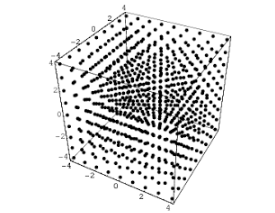# Lattice Based CryptographyLattice Based Cryptography

## Cryptography

Before we dive into all fun juicy topic of lattice based cryptography we must know about cryptography. Cryptography is study and practice of technique used to maintain confidentiality of data.In layman's term cryptography is about constructing and analysing the protocol which prevents a third person from reading a private message or data maintaining the confidentiality. Cryptography exist on the principles of mathematics and computer science.

## What is a Lattice?Lattice is a periodic grid of m dimensional integer vectors. Lattice can be finitely represented using bassis B where B is set of m dimensional linearly independent integer vector having n number entries.

B={b1,b2,b3…..,bn}

A lattice can be represented by organizing bassis B vectors in nxm matrix.

A={b1,b2,b3…..bn}nxm

## Hard Problem: Short integer Solution Domains

Now that we know what is a lattice to create a cryptography out of it we need a hard problem on lattices

Ajatai in 1996 introduced a problem named Short Integer Solution Problem which is a very hard problem to solve in instances of average case as well as worst case.

Here,

Znxmq= n dimensional integer vectors modulo q

The goal here is to find non-trivial z such that z ∈{01}m where m is number of vectors such that Anxm z1xm=0

Solving this problem on a lattie need exponential times dimensions runtime which makes this problem really hard to solve even on quantum computers!!!

## Creating Collision-Resistant Hash Function

Using the short integer solution problem we can create hash function.

Here set m > nlog2q and define a shrinking function f A:{0,1}m ∈Zqnxm where fA = Anxmx1xm and all arithmetics are mod qx is a binary string of length m.

Now you must be thinking “How this is a collision resistant function?”

Lets,

Anxm x1xm = Anxm x̄1xm = 0

Anxm x1xm - A x̄ 1xm=0

Anxm(x-x̄)1xm = 0

Which is similar to Anxm z1xm=0 so z1xm= (x-x̄)1xm which same as finding the solution to short integer problem introduced by Ajtai which needs runtime of exponential times in dimensions.

## Why should we use Lattice?

• The schemes tend to be quite efficient they involve linear operations rather than complex exponentiation on large numbers used algorithms like RSA.
• Can resist attacks made using a quantum computer.
• We can do almost all the things done by the currently existing cryptographic algorithms.

Adding to above features lattice based cryptography provides solution for a lot of new application like fully homomorphic encryption/signature ,Attribute based encryption ,Functional encryption and program obfuscation in such a way that it can be executed on a normal computers but cannot reverse engineer. Lattice based cryptography is clearly the future of cryptography with its wide variety of applications and features.